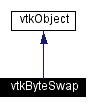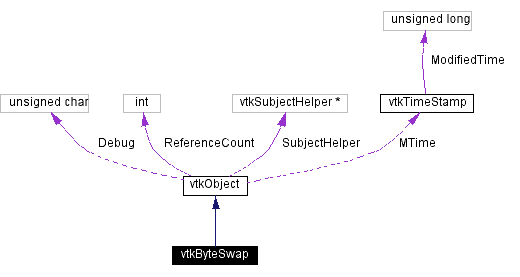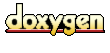Main Page   Class Hierarchy   Alphabetical List   Compound List   File List   Compound Members   File Members   Related Pages

# vtkByteSwap Class Reference

perform machine dependent byte swapping. More...

`#include <vtkByteSwap.h>`

Inheritance diagram for vtkByteSwap:[legend]
Collaboration diagram for vtkByteSwap:[legend]
List of all members.

## Public Methods

virtual const char * GetClassName ()
virtual int IsA (const char *type)

## Static Public Methods

vtkByteSwap * New ()
int IsTypeOf (const char *type)
vtkByteSwap * SafeDownCast (vtkObject *o)
void Swap2LE (short *s)
void Swap4LE (char *c)
void Swap4LE (float *p)
void Swap4LE (int *i)
void Swap4LE (unsigned long *i)
void Swap4LE (long *i)
void Swap4LERange (char *c, int num)
void Swap4LERange (unsigned char *c, int num)
void Swap4LERange (float *p, int num)
void Swap4LERange (int *i, int num)
void Swap4LERange (unsigned long *i, int num)
void Swap4BE (char *c)
void Swap4BE (float *p)
void Swap4BE (int *i)
void Swap4BE (unsigned long *i)
void Swap4BERange (char *c, int num)
void Swap4BERange (float *p, int num)
void Swap4BERange (int *i, int num)
void Swap4BERange (unsigned long *i, int num)
void SwapWrite4BERange (char *c, int num, FILE *fp)
void SwapWrite4BERange (float *p, int num, FILE *fp)
void SwapWrite4BERange (int *i, int num, FILE *fp)
void SwapWrite4BERange (unsigned long *i, int num, FILE *fp)
void SwapWrite4BERange (char *c, int num, ostream *fp)
void SwapWrite4BERange (float *p, int num, ostream *fp)
void SwapWrite4BERange (int *i, int num, ostream *fp)
void SwapWrite4BERange (unsigned long *i, int num, ostream *fp)
void Swap2BE (short *s)
void Swap2BERange (char *c, int num)
void Swap2BERange (short *i, int num)
void Swap2LERange (char *c, int num)
void Swap2LERange (short *i, int num)
void SwapWrite2BERange (char *c, int num, FILE *fp)
void SwapWrite2BERange (short *i, int num, FILE *fp)
void SwapWrite2BERange (char *c, int num, ostream *fp)
void SwapWrite2BERange (short *i, int num, ostream *fp)
void SwapVoidRange (void *buffer, int numWords, int wordSize)

## Protected Methods

vtkByteSwap ()
~vtkByteSwap ()
vtkByteSwap (const vtkByteSwap &)
void operator= (const vtkByteSwap &)

## Detailed Description

perform machine dependent byte swapping.

Date:
2000/12/10 20:08:06
Revision:
1.38

vtkByteSwap is used by other classes to perform machine dependent byte swapping. Byte swapping is often used when reading or writing binary files.

Definition at line 56 of file vtkByteSwap.h.

## Constructor & Destructor Documentation

 vtkByteSwap::vtkByteSwap ( ) ` [inline, protected]`
 Definition at line 157 of file vtkByteSwap.h.

 vtkByteSwap::~vtkByteSwap ( ) ` [inline, protected]`
 Definition at line 158 of file vtkByteSwap.h.

 vtkByteSwap::vtkByteSwap ( const vtkByteSwap & ) ` [inline, protected]`
 Definition at line 159 of file vtkByteSwap.h.

## Member Function Documentation

 vtkByteSwap* vtkByteSwap::New ( ) ` [static]`
 Create an object with Debug turned off, modified time initialized to zero, and reference counting on. Reimplemented from vtkObject.

 virtual const char* vtkByteSwap::GetClassName ( ) ` [virtual]`
 Return the class name as a string. This method is defined in all subclasses of vtkObject with the vtkTypeMacro found in vtkSetGet.h. Reimplemented from vtkObject.

 int vtkByteSwap::IsTypeOf ( const char * type ) ` [static]`
 Return 1 if this class type is the same type of (or a subclass of) the named class. Returns 0 otherwise. This method works in combination with vtkTypeMacro found in vtkSetGet.h. Reimplemented from vtkObject.

 virtual int vtkByteSwap::IsA ( const char * type ) ` [virtual]`
 Return 1 if this class is the same type of (or a subclass of) the named class. Returns 0 otherwise. This method works in combination with vtkTypeMacro found in vtkSetGet.h. Reimplemented from vtkObject.

 vtkByteSwap* vtkByteSwap::SafeDownCast ( vtkObject * o ) ` [static]`
 Will cast the supplied object to vtkObject* is this is a safe operation (i.e., a safe downcast); otherwise NULL is returned. This method is defined in all subclasses of vtkObject with the vtkTypeMacro found in vtkSetGet.h. Reimplemented from vtkObject.

 void vtkByteSwap::Swap2LE ( short * s ) ` [static]`
 Swap 2 byte word to be LE.

 void vtkByteSwap::Swap4LE ( char * c ) ` [static]`
 Swap four byte word to be LE. Referenced by Swap4LE().

 void vtkByteSwap::Swap4LE ( float * p ) ` [inline, static]`
 Definition at line 68 of file vtkByteSwap.h.

 void vtkByteSwap::Swap4LE ( int * i ) ` [inline, static]`
 Definition at line 69 of file vtkByteSwap.h.

 void vtkByteSwap::Swap4LE ( unsigned long * i ) ` [inline, static]`
 Definition at line 70 of file vtkByteSwap.h.

 void vtkByteSwap::Swap4LE ( long * i ) ` [inline, static]`
 Definition at line 71 of file vtkByteSwap.h.

 void vtkByteSwap::Swap4LERange ( char * c, int num ) ` [static]`
 Swap bunch of bytes to be LE. Num is the number of four byte words to swap. Referenced by Swap4LERange().

 void vtkByteSwap::Swap4LERange ( unsigned char * c, int num ) ` [inline, static]`
 Definition at line 77 of file vtkByteSwap.h.

 void vtkByteSwap::Swap4LERange ( float * p, int num ) ` [inline, static]`
 Definition at line 79 of file vtkByteSwap.h.

 void vtkByteSwap::Swap4LERange ( int * i, int num ) ` [inline, static]`
 Definition at line 81 of file vtkByteSwap.h.

 void vtkByteSwap::Swap4LERange ( unsigned long * i, int num ) ` [inline, static]`
 Definition at line 83 of file vtkByteSwap.h.

 void vtkByteSwap::Swap4BE ( char * c ) ` [static]`
 Swap four byte word to be BE. Referenced by Swap4BE().

 void vtkByteSwap::Swap4BE ( float * p ) ` [inline, static]`
 Definition at line 89 of file vtkByteSwap.h.

 void vtkByteSwap::Swap4BE ( int * i ) ` [inline, static]`
 Definition at line 90 of file vtkByteSwap.h.

 void vtkByteSwap::Swap4BE ( unsigned long * i ) ` [inline, static]`
 Definition at line 91 of file vtkByteSwap.h.

 void vtkByteSwap::Swap4BERange ( char * c, int num ) ` [static]`
 Swap bunch of bytes to be BE. Num is the number of four byte words to swap. Referenced by Swap4BERange().

 void vtkByteSwap::Swap4BERange ( float * p, int num ) ` [inline, static]`
 Definition at line 97 of file vtkByteSwap.h.

 void vtkByteSwap::Swap4BERange ( int * i, int num ) ` [inline, static]`
 Definition at line 99 of file vtkByteSwap.h.

 void vtkByteSwap::Swap4BERange ( unsigned long * i, int num ) ` [inline, static]`
 Definition at line 101 of file vtkByteSwap.h.

 void vtkByteSwap::SwapWrite4BERange ( char * c, int num, FILE * fp ) ` [static]`
 Swap bunch of bytes to BE. Num is the number of four byte words to swap. The results are written out to prevent having to keep the swapped copy in memory. Referenced by SwapWrite4BERange().

 void vtkByteSwap::SwapWrite4BERange ( float * p, int num, FILE * fp ) ` [inline, static]`
 Definition at line 109 of file vtkByteSwap.h.

 void vtkByteSwap::SwapWrite4BERange ( int * i, int num, FILE * fp ) ` [inline, static]`
 Definition at line 111 of file vtkByteSwap.h.

 void vtkByteSwap::SwapWrite4BERange ( unsigned long * i, int num, FILE * fp ) ` [inline, static]`
 Definition at line 113 of file vtkByteSwap.h.

 void vtkByteSwap::SwapWrite4BERange ( char * c, int num, ostream * fp ) ` [static]`

 void vtkByteSwap::SwapWrite4BERange ( float * p, int num, ostream * fp ) ` [inline, static]`
 Definition at line 117 of file vtkByteSwap.h.

 void vtkByteSwap::SwapWrite4BERange ( int * i, int num, ostream * fp ) ` [inline, static]`
 Definition at line 119 of file vtkByteSwap.h.

 void vtkByteSwap::SwapWrite4BERange ( unsigned long * i, int num, ostream * fp ) ` [inline, static]`
 Definition at line 121 of file vtkByteSwap.h.

 void vtkByteSwap::Swap2BE ( short * s ) ` [static]`
 Swap 2 byte word to BE.

 void vtkByteSwap::Swap2BERange ( char * c, int num ) ` [static]`
 Swap bunch of bytes to BE. Num is the number of two byte words to swap. Referenced by Swap2BERange().

 void vtkByteSwap::Swap2BERange ( short * i, int num ) ` [inline, static]`
 Definition at line 131 of file vtkByteSwap.h.

 void vtkByteSwap::Swap2LERange ( char * c, int num ) ` [static]`
 Swap bunch of bytes to LE. Num is the number of two byte words to swap. Referenced by Swap2LERange().

 void vtkByteSwap::Swap2LERange ( short * i, int num ) ` [inline, static]`
 Definition at line 137 of file vtkByteSwap.h.

 void vtkByteSwap::SwapWrite2BERange ( char * c, int num, FILE * fp ) ` [static]`
 Swap bunch of bytes to BE. Num is the number of two byte words to swap. The results are written out to prevent having to keep the swapped copy in memory. Referenced by SwapWrite2BERange().

 void vtkByteSwap::SwapWrite2BERange ( short * i, int num, FILE * fp ) ` [inline, static]`
 Definition at line 145 of file vtkByteSwap.h.

 void vtkByteSwap::SwapWrite2BERange ( char * c, int num, ostream * fp ) ` [static]`

 void vtkByteSwap::SwapWrite2BERange ( short * i, int num, ostream * fp ) ` [inline, static]`
 Definition at line 149 of file vtkByteSwap.h.

 void vtkByteSwap::SwapVoidRange ( void * buffer, int numWords, int wordSize ) ` [static]`
 Swaps the bytes of a buffer. Uses an arbitrary word size, but assumes the word size is divisible by two.

 void vtkByteSwap::operator= ( const vtkByteSwap & ) ` [inline, protected]`
 Definition at line 160 of file vtkByteSwap.h.

The documentation for this class was generated from the following file:

Generated on Wed Nov 21 12:46:38 2001 for VTK by1.2.11.1 written by Dimitri van Heesch, © 1997-2001